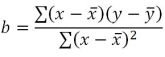# SQL Server SLOPE function

SLOPE

Updated: 24 September 2010

Note: This documentation is for the SQL2008 (and later) version of this XLeratorDB function, it is not compatible with SQL Server 2005.

Use the multi-input aggregate SLOPE function to calculate the slope of the linear regression through the data points in the known x-values and y-values. The slope is the vertical distance divided by the horizontal distance between any two points on the line, which is the rate of change along the regression line. The equation for the slope of the regression line is:Syntax
SELECT [wctStatistics].[wct].[SLOPE] (
,<@Known_y, float,>
,<@Known_x, float,>)
Arguments

@Known_y
the y-values to be used in the SLOPE calculation. @Known_y is an expression of type float or of a type that can be implicitly converted to float.
@Known_x
the x-values to be used in the SLOPE calculation. @Known_x is an expression of type float or of a type that can be implicitly converted to float.
Return Types
float
Remarks
·         If the number of y-data points is not equal to the number of x-data points, SLOPE will return an error.
·         SLOPE is an AGGREGATE function and follows the same conventions as all other AGGREGATE functions in SQL Server.
Examples
In this example, we calculate the slope for a single set of x- and y-values
SELECT wct.SLOPE(y, x) as SLOPE
FROM (
SELECT 0.75, 1 UNION ALL
SELECT 2.5, 2 UNION ALL
SELECT 6.75, 3 UNION ALL
SELECT 10, 4
) n(x,y)

This produces the following result
SLOPE
----------------------
0.305489260143198

(1 row(s) affected)
In this example, we will populate some temporary table with some historical financial information and then calculate the slope. First, create the table and put some data in it:
CREATE TABLE #c(
SYM         NVARCHAR(5),
YE          BIGINT,
REV         FLOAT,
GPROF       FLOAT,
OPINC       FLOAT,
NETINC      FLOAT
)

INSERT INTO #c VALUES('YHOO',2009,6460.32,3588.57,386.69,597.99)
INSERT INTO #c VALUES('YHOO',20,72.5,4185.14,12.96,418.92)
INSERT INTO #c VALUES('YHOO',2007,6969.27,4130.52,695.41,639.16)
INSERT INTO #c VALUES('YHOO',2006,6425.68,3749.96,940.97,751.39)
INSERT INTO #c VALUES('YHOO',2005,5257.67,3161.47,1107.73,1896.23)
INSERT INTO #c VALUES('GOOG',2009,23650.56,14806.45,8312.19,6520.45)
INSERT INTO #c VALUES('GOOG',20,21795.55,13174.04,5537.21,4226.86)
INSERT INTO #c VALUES('GOOG',2007,16593.99,9944.9,54.44,4203.72)
INSERT INTO #c VALUES('GOOG',2006,10604.92,6379.89,3550,3077.45)
INSERT INTO #c VALUES('GOOG',2005,6138.56,3561.47,2017.28,1465.4)
INSERT INTO #c VALUES('MSFT',2010,62484,509,24167,18760)
INSERT INTO #c VALUES('MSFT',2009,58437,46282,21225,14569)
INSERT INTO #c VALUES('MSFT',20,60420,48822,22271,17681)
INSERT INTO #c VALUES('MSFT',2007,51122,40429,18438,14065)
INSERT INTO #c VALUES('MSFT',2006,44282,36632,16064,12599)
INSERT INTO #c VALUES('ORCL',2010,26820,21056,9062,6135)
INSERT INTO #c VALUES('ORCL',2009,23252,18458,8321,5593)
INSERT INTO #c VALUES('ORCL',20,22430,17449,7844,5521)
INSERT INTO #c VALUES('ORCL',2007,17996,13805,5974,4274)
INSERT INTO #c VALUES('ORCL',2006,14380,11145,4736,3381)
INSERT INTO #c VALUES('SAP',2009,10672,6980,2588,1748)
INSERT INTO #c VALUES('SAP',20,11575,7370,2701,1847)
INSERT INTO #c VALUES('SAP',2007,10256,6631,2698,1906)
INSERT INTO #c VALUES('SAP',2006,9393,6064,2578,1871)
INSERT INTO #c VALUES('SAP',2005,8509,5460,2337,1496)
Now, calculate the slope of the revenue (REV) againt the year (YE) for each company (SYM)
SELECT SYM
,wct.SLOPE(REV,YE) as SLOPE
FROM #c
GROUP BY SYM
This produces the following result.
SYM   SLOPE
----- ----------------------
GOOG 4621.46300000191
MSFT 4371.9
ORCL 3013.6
SAP  650.8
YHOO 318.811999999285
In this example, we will calculate the slope of the operating income (OPINC) against the revenue (REV)
SELECT SYM
,wct.SLOPE(OPINC,REV) as SLOPE
FROM #c
GROUP BY SYM
This produces the following result.
SYM   SLOPE
----- ----------------------
GOOG 0.300273324369858
MSFT 0.420268251175422
ORCL 0.365274953269938
SAP  0.106096856457427
YHOO -0.4472757613674
Let’s say we wanted to perform the same analysis as above, but we only want to return the results where the slope is positive.
SELECT SYM
,wct.SLOPE(OPINC,REV) as SLOPE
FROM #c
GROUP BY SYM
HAVING wct.SLOPE(OPINC,REV) > 0
This produces the following result.
SYM   SLOPE
----- ----------------------
GOOG 0.300273324369858
MSFT 0.420268251175422
ORCL 0.365274953269938
SAP  0.106096856457427Copyright 2008-2023 Westclintech LLC         Privacy Policy        Terms of Service